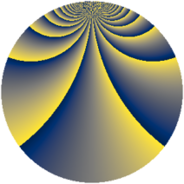# Properties

 Label 2001.2.bvLevel $2001$ Weight $2$ Character orbit 2001.bv Rep. character $\chi_{2001}(2,\cdot)$ Character field $\Q(\zeta_{308})$ Dimension $28320$ Sturm bound $480$

# Related objects

## Defining parameters

 Level: $$N$$ $$=$$ $$2001 = 3 \cdot 23 \cdot 29$$ Weight: $$k$$ $$=$$ $$2$$ Character orbit: $$[\chi]$$ $$=$$ 2001.bv (of order $$308$$ and degree $$120$$) Character conductor: $$\operatorname{cond}(\chi)$$ $$=$$ $$2001$$ Character field: $$\Q(\zeta_{308})$$ Sturm bound: $$480$$

## Dimensions

The following table gives the dimensions of various subspaces of $$M_{2}(2001, [\chi])$$.

Total New Old
Modular forms 29280 29280 0
Cusp forms 28320 28320 0
Eisenstein series 960 960 0

## Trace form

 $$28320q - 108q^{3} - 252q^{4} - 126q^{6} - 180q^{7} - 126q^{9} + O(q^{10})$$ $$28320q - 108q^{3} - 252q^{4} - 126q^{6} - 180q^{7} - 126q^{9} - 232q^{10} - 110q^{12} - 252q^{13} - 120q^{15} - 564q^{16} - 110q^{18} - 224q^{19} - 82q^{21} - 560q^{22} - 48q^{24} + 260q^{25} - 246q^{27} - 256q^{30} - 200q^{31} - 210q^{33} - 252q^{34} - 166q^{36} - 200q^{37} - 48q^{39} - 240q^{40} - 126q^{42} - 296q^{43} - 240q^{45} - 208q^{46} - 158q^{48} + 164q^{49} - 126q^{51} - 340q^{52} - 162q^{54} - 188q^{55} - 4q^{58} - 124q^{60} - 360q^{61} - 126q^{63} - 504q^{64} - 338q^{66} - 364q^{67} - 142q^{69} - 736q^{70} - 44q^{72} - 180q^{73} - 84q^{75} + 24q^{76} + 174q^{78} - 264q^{79} + 118q^{81} - 212q^{82} - 100q^{84} - 60q^{85} - 352q^{87} - 720q^{88} + 120q^{90} - 392q^{91} - 280q^{93} - 220q^{94} - 168q^{96} - 208q^{97} + 164q^{99} + O(q^{100})$$

## Decomposition of $$S_{2}^{\mathrm{new}}(2001, [\chi])$$ into newform subspaces

The newforms in this space have not yet been added to the LMFDB.